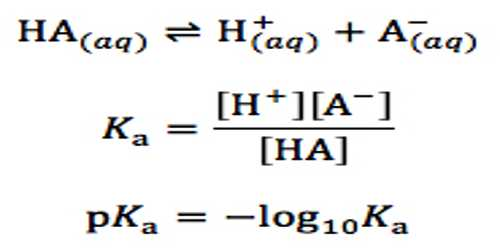# pH of Solutions of very Weak Acids

pH of solutions of very weak acids

If the acid is very weak then the extent of dissociation will be very small and for all practical purposes [HA] in the equation may be taken as the initial concentration of the acid. As [H+] = [A] equation reduces to

[H+]2 = Ka [HA]

so that, [H+] = √{Ka [HA]} … … … (1)

This relation may be used to make approximate calculations of pH of solutions of very weak and moderately weak acids. If the pH of the solution of a weak acid is known an approximate value of its Ka may be calculated. The value of Ka may also be determined by a titration curve.Example: Calculate the pH of a solution of n-butyric acid concentration 0.12 mol L-1 (Ka of the acid = 1.515 x 10-5 mol L-1).

Solution: Using the relation (1), [H+] = √{Ka [HA]}

= √[0.12×1.515×10-5]

= 1.66 x 10-3

pH = – log (1.66 x 10-3) = 2.78.

pH is a measure of the concentration of hydrogen ions in a solution. Strong acids like hydrochloric acid at the sort of concentrations you normally use in the lab have a pH around 0 to 1. The lower the pH, the higher the concentration of hydrogen ions in the solution./sub